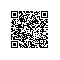# Android For JNI(五)——C语言多级指针，结构体，联合体，枚举，自定义类型

## 一.多级指针

#include <stdio.h>
#include <stdlib.h>

main(){

int i = 3;
//一级
int* p = &i;

//二级，保存一级指针
int** w = &p;

//三级 保存二级指针
int*** e = &w;

printf("%d\n",*(*w));
printf("%d\n",***e);
//让窗口停留
int age ;
scanf("%d",&age);

}## 二.结构体

#include <stdio.h>
#include <stdlib.h>

struct student{

int age;
char sex;
};

main(){

//定义结构体
struct student stu = {20,170,'g'};

printf("%d\n",stu.age);
printf("%d\n",sizeof(stu));

//让窗口停留
int age ;
scanf("%d",&age);

}### 结构体函数指针#include <stdio.h>
#include <stdlib.h>

void study(){
printf("结构体函数\n");
}

struct student{

int age;
char sex;

void (*studentp)();
};

main(){

//定义结构体
struct student stu = {20,170,'g', study };

// printf("%d\n",stu.age);
// printf("%d\n",sizeof(stu));

stu.studentp();

//让窗口停留
int age ;
scanf("%d",&age);

}



       struct student* stup = &stu;
(*stup).study();
//引用这个指针的属性
stup->study();

### 三.联合体

#include <stdio.h>
#include <stdlib.h>

main(){
//定义联合体
union{int i ; short j ;char k}un;
//他跟结构体的用法类似
un.i = 30;
printf("%d\n",un.i);
//打印长度
printf("%d\n",sizeof(un));

//让窗口停留
int age ;
scanf("%d",&age);

}#include <stdio.h>
#include <stdlib.h>

main(){
//定义联合体
union{int i ; short j ;char k}un;
//他跟结构体的用法类似
un.i = 30;
printf("%d\n",un.i);
un.j = 20;
printf("%d\n",un.i);
//打印长度
printf("%d\n",sizeof(un));

//让窗口停留
int age ;
scanf("%d",&age);

}i = 20了，那是因为联合体每次只能存放一个数据，有值的话就替换

## 四.枚举

#include <stdio.h>
#include <stdlib.h>

enum WeekDay
{
Monday,Tuesday,Wednesday,Thursday,Friday,Saturday,Sunday
};

int main(void)
{
//int day;
enum WeekDay day = Sunday;
printf("%d\n",day);
system("pause");
return 0;
}


## 五.自定义类型

#include <stdio.h>
#include <stdlib.h>
//自定义类型
typedef int hello;

main(){

hello i = 3;
printf("%d\n",i);

//让窗口停留
int age ;
scanf("%d",&age);

}使用钉钉扫一扫加入圈子
+ 订阅